Author: Oscar Cronquist Article last updated on December 08, 2017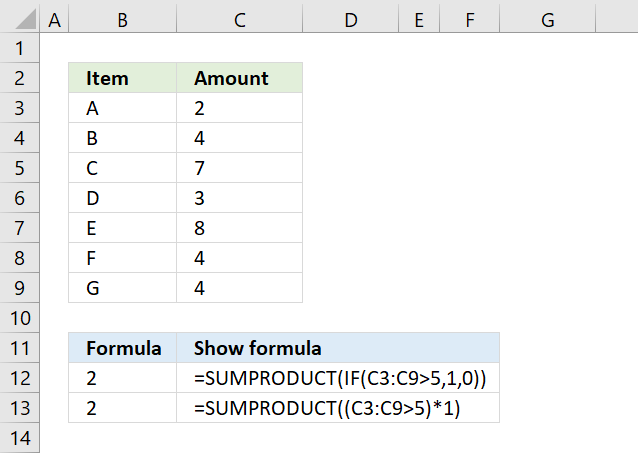You don't need to use the IF function in a SUMPRODUCT function, it is enough to use a logical expression. For example, the array formula above in cell B12 counts all cells in C3:C9 that are above 5 using an IF function.

=SUMPRODUCT(IF(C3:C9>5,1,0))

The first argument in the IF function is a logical expression, use that in your SUMPRODUCT formula. The formula in B13 does the same thing as in B12.

=SUMPRODUCT((C3:C9>5)*1)

You need to tell Excel the order of calculation, in other words, make sure you calculate C3:C9>5 before you multiply by 1. To do that use parentheses.

(C3:C9>5)*1

The larger than comparison operator compares each value in C3:C9 if larger than 5. It returns an array the same size as C3:C9 containing boolean values, TRUE or FALSE.

{FALSE; FALSE; TRUE; FALSE; TRUE; FALSE; FALSE}*1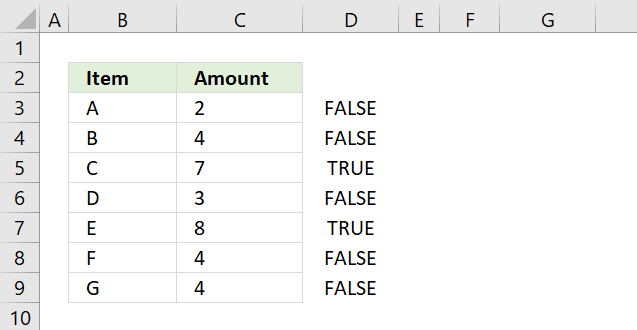The picture above shows the array in column D, value 7 and 8 are larger than 5.

The SUMPRODUCT function can't sum boolean values so multiply (using the asterisk *) the logical expression by 1 to convert the boolean values (TRUE and FALSE) to numbers (1 or 0).

{0;0;1;0;1;0;0}

The asterisk also has another advantage, you can enter the SUMPRODUCT formula as a regular formula.

### Combine SUMPRODUCT and IF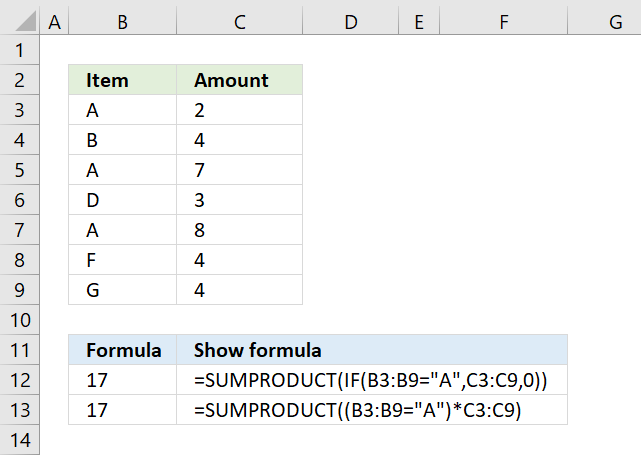The picture above demonstrates an IF function that checks if a condition is met and if TRUE returns a value in a corresponding cell.

There is no need for the IF function in the above example, simply use the logical expression and multiply with the values you want to use, demonstrated in cell B13.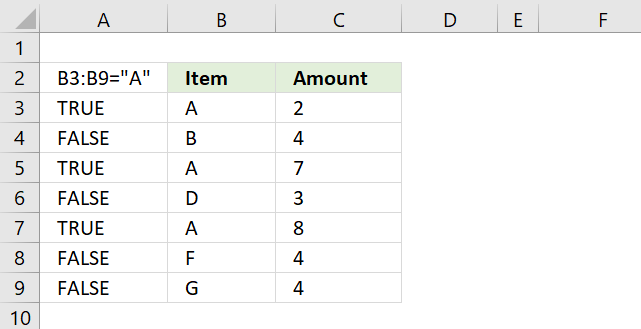B3:B9="A" returns {TRUE;FALSE;TRUE;FALSE;TRUE;FALSE;FALSE} displayed in column A in image above.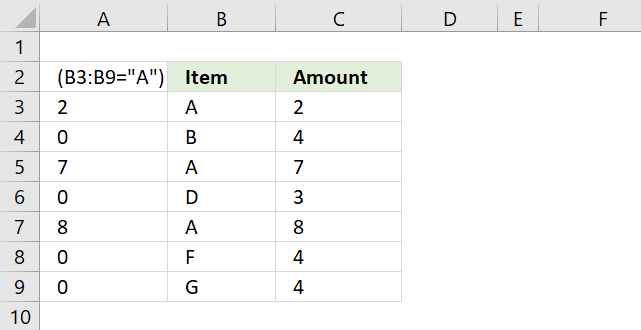(B3:B9="A")*C3:C9 returns this array: {2;0;7;0;8;0;0} shown in column A.Lastly, the SUMPRODUCT function sums all the numbers in the array returning 17 in cell B12.

2 + 7 + 8 = 17.